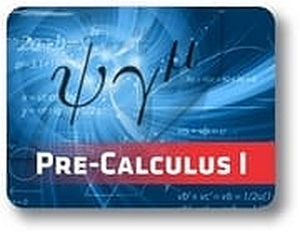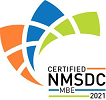Red Comet## Pre-Calculus - Semester - 1

#### Course Price: \$285.00## Course Details:

This course builds on Algebra I & II and lays a solid foundation for a future course in Calculus. Student learns about several important concepts like Matrices, Sequences & Series and Complex Numbers. This course first provides a detailed introduction to the study of Trigonometry and later helps the student learn advanced concepts in this topic. With a firm understanding of Trigonometry and various concepts in Algebra, the student is well-prepared to handle the challenges of Calculus.

Note: This course is not designed for ELL (English Language Learners) students. ELL students may enroll in this course ONLY if they have adequate mentor support at their home school and are able to fulfill all course requirements.

## Syllabus:

Section 1: Matrices

• Introduction to Matrices
• Types of Matrices
• Add and Subtract Matrices
• Multiplication of Matrices
• Solving Matrix Equations
• Determinant of Matrix
• Inverse of Matrices
• Transformation of Matrices
• Examples of Transformations

Section 2: Sequences and Series

• Introduction to Sequences
• Fibonacci Sequence
• Types of Sequences
• Examples of Sequences
• Introduction to Series
• Arithmetic and Geometric Series
• Partial Sums
• Infinite Series

Section 3: introduction to Trigonometry

• Overview of Trigonometry
• Conversions
• Trigonometry Basics
• Trigonometric Identities
• Special Triangles and Ratios
• Trigonometry and Circles

Section 4: Concepts of Trigonometry

• Properties of Trigonometric Functions
• Sine Function
• Cosine Function
• Sine Cosine Relation
• Inverse Trigonometric Functions

Section 5: Complex Numbers

• Introduction to Imaginary Numbers
• Adding and Subtracting Complex Numbers
• Conjugate Complex Number
• Multiplying and Dividing Complex Numbers
• Absolute Value of a Complex Number
• Introduction to Complex Plane
• Finding Values on a Complex Plane
• Introduction to Polar Form
• Polar Form to Rectangular Form
• Circle, Line – Polar Form

### Accreditation & Approvals